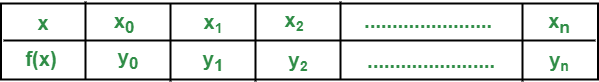Open in App
Not now

# Difference between Interpolation and Extrapolation

• Last Updated : 21 Oct, 2021

1. Interpolation :
Interpolation refers to the estimation of a single value from two known values which are given from a sequence of values.

2. Extrapolation :
Extrapolation refers to the estimation of a single value from the given sequence of values where the background of values is known at certain times.

More precisely, we can define interpolation and extrapolation is as follows:

Suppose that we are given the following tabulated values of the function f(x) corresponding to a discrete set of values of x:Interpolation is the process of finding the value of f(x) corresponding to any untabulated value of x between x0 and xn. The process of finding the value of f(x) for some value of x outside the given range  [x0, xn] is called extrapolation. It assumes that the behaviour of f(x) outside the given range is identical to the behaviour of f(x) inside the given range and this may not always be valid.

Difference between Interpolation and Extrapolation is as follows:

My Personal Notes arrow_drop_up
Related Articles